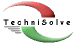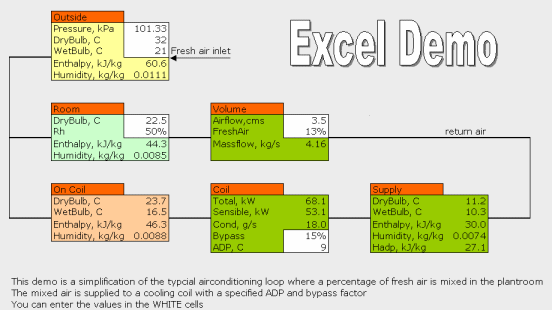#TechniSolve Software

### Software for the air conditioning, ventilation and refrigeration industry

#### Using the TechniSolve Psychrometric Library in Excel

Up ] ACSpec ] AirState ] DutyCalc ] [ Excel ] Chart Plotter ] TextBook ]

One of the great benefits of using a Dynamic Link Library (DLL) is the ability to share code.  I have written many refrigeration and airconditioning programs that need psychrometric calculations so it would make sense to used a shared resource.  By writing the Psy42.dll library, all of my programs use this common library.This saves programming time, memory space, ensures repeatability across the program range and also gives you, the user, access to a very powerful psychrometric number cruncher.  By using this DLL, you can now do psychrometric calculations in Excel without writing any code. The Excel demo that we have supplied shows how.

Firstly, let's understand what a DLL is.  Normal programs that you run have the extension EXE.  These are normally  executable programs that you would be able to run from the Windows menu.  The DLL is a compiled group of functions that can be used by programs but not run as stand-alone programs.  Microsoft use this method extensively within their operating system, probably for the same reasons sited above.

This document gives you all the information that you need to be able to use the psychrometrics library effectively.

#### Our Psy42.dll Psychrometric library exports the following functions:

function AtmPressure(Z: Double): Double; stdcall;
function Altitude(P: Double): Double; stdcall;
function HeatCapacity(W: Double): Double; stdcall;
function SatPressure(t: Double): Double; stdcall;
function LatentHeat(t: Double): Double; stdcall;
function DynViscosity(t: Double): Double; stdcall;
function Conductivity(t: Double): Double; stdcall;
function DryBulb(const Patm, arg1, arg2: Double; const Cmb: TAirCmb): Double; stdcall;
function WetBulb(const Patm, arg1, arg2: Double; const Cmb: TAirCmb): Double; stdcall;
function Enthalpy(const Patm, arg1, arg2: Double; const Cmb: TAirCmb): Double; stdcall;
function Humidity(const Patm, arg1, arg2: Double; const Cmb: TAirCmb): Double; stdcall;
function DewPoint(const Patm, arg1, arg2: Double; const Cmb: TAirCmb): Double; stdcall;
function RelHumidity(const Patm, arg1, arg2: Double; const Cmb: TAirCmb): Double; stdcall;
function VapPressure(const Patm, arg1, arg2: Double; const Cmb: TAirCmb): Double; stdcall;
function Entropy(const Patm, arg1, arg2: Double; const Cmb: TAirCmb): Double; stdcall;
function Density(const Patm, arg1, arg2: Double; const Cmb: TAirCmb): Double; stdcall;
function QEnthalpy(const Patm: Double; arg: String): Double;

The above is the actual Pascal interface that I show here just to give you the complete picture.  If you look at the PsyDemo.xls file attached, you will see that I have done some of the work for you in putting this into Excel.

Declare Function AtmPressure Lib "Psy42" (ByVal z As Double) As Double
Declare Function Altitude Lib "Psy42" (ByVal p As Double) As Double
Declare Function HeatCapacity Lib "Psy42" (ByVal W As Double) As Double
Declare Function SatPressure Lib "Psy42" (ByVal t As Double) As Double
Declare Function LatentHeat Lib "Psy42" (ByVal t As Double) As Double
Declare Function DynViscosity Lib "Psy42" (ByVal t As Double) As Double
Declare Function Conductivity Lib "Psy42" (ByVal t As Double) As Double
Declare Function DryBulb Lib "Psy42" (ByVal Patm As Double, ByVal arg1 As Double, ByVal arg2 As Double, ByVal Opt As Byte) As Double
Declare Function WetBulb Lib "Psy42" (ByVal Patm As Double, ByVal arg1 As Double, ByVal arg2 As Double, ByVal Opt As Byte) As Double
Declare Function Enthalpy Lib "Psy42" (ByVal Patm As Double, ByVal arg1 As Double, ByVal arg2 As Double, ByVal Opt As Byte) As Double
Declare Function Humidity Lib "Psy42" (ByVal Patm As Double, ByVal arg1 As Double, ByVal arg2 As Double, ByVal Opt As Byte) As Double
Declare Function DewPoint Lib "Psy42" (ByVal Patm As Double, ByVal arg1 As Double, ByVal arg2 As Double, ByVal Opt As Byte) As Double
Declare Function RelHumidity Lib "Psy42" (ByVal Patm As Double, ByVal arg1 As Double, ByVal arg2 As Double, ByVal Opt As Byte) As Double
Declare Function VapPressure Lib "Psy42" (ByVal Patm As Double, ByVal arg1 As Double, ByVal arg2 As Double, ByVal Opt As Byte) As Double
Declare Function Entropy Lib "Psy42" (ByVal Patm As Double, ByVal arg1 As Double, ByVal arg2 As Double, ByVal Opt As Byte) As Double
Declare Function Density Lib "Psy42" (ByVal Patm As Double, ByVal arg1 As Double, ByVal arg2 As Double, ByVal Opt As Byte) As Double

If you compare these with the Pascal code, you will find that they are very similar.  You can find the 2nd set in the PsyDemo.xls file if you open the Visual Basic Editor and look into Module1.

Once these are in place, you can do Psychrometrics in an Excel workbook by simply using the functions like you would with any of the standard Excel functions.  So, in any cell, type in the command

=Enthalpy(Pressure, DryBulb, WetBulb, 0) and you will see the result in the cell.

The option in the example above is 0.  This is the code that tells the library to assume DB and WB as inputs.  You can use any of the options that we have programmed by setting the option code as shown below ( numbered from 0 ).

dbwb, dbHa, dbWa, dbRh, dbTd, dbPv, dbZa,
wbWa, wbRh, wbTd, wbPv, wbPs,
HaWa, HaRh, HaTd, HaPv, HaPs,
ZaWa, ZaRh, ZaTd, ZaPv, ZaPs,
WaRh, WaPs, WaRo,
RhTd, RhPv, RhPs,
TdPs, PvPs

If, for example, you want to calculate the humidity given DryBulb and Enthalpy, enter the function

=Humidity(Pressure, DryBulb, Enthalpy, 1)

How's that for convenience?

...

You can here but you must have installed one of our airconditioning programs for the dynamic link library to be installed.